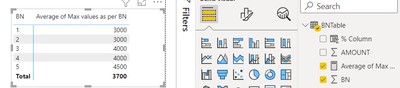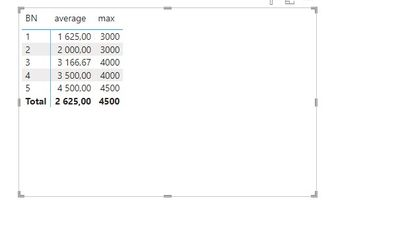cancel
Showing results for
Did you mean:Helper V

## Maximum and Average

Measure to get the maximum amount for each BN and then average the maximum amount.

Below is the sample data for reference.

 BN AMOUNT 1 1000 1 2000 1 3000 2 1000 3 2000 4 3000 2 3000 3 4000 4 4000 1 500 3 3500 5 4500

2 ACCEPTED SOLUTIONSSuper User

I think you are looking for this:

``Average of Max values as per BN = AVERAGEX(SUMMARIZE(BNTable,BNTable[BN],"MaxBN",MAX(BNTable[AMOUNT])),[MaxBN])``

It gives an outcome of 3700 with the given data.Super User

You can visualise it like this:5 REPLIES 5Super User

I think you are looking for this:

``Average of Max values as per BN = AVERAGEX(SUMMARIZE(BNTable,BNTable[BN],"MaxBN",MAX(BNTable[AMOUNT])),[MaxBN])``

It gives an outcome of 3700 with the given data.Helper V

Thanks.

this is the result i was looking for.

Now, what if i would like to get with code "BS" only.

Below sample refer.

 BN AMOUNT Code 1 1000 BS 1 2000 BS 1 3000 H 2 1000 BS 3 2000 BS 4 3000 BS 2 3000 H 3 4000 BS 4 4000 BS 1 500 BS 3 3500 H 5 4500 BSHelper I

Hi !

average = AVERAGE('Table'[amount])
max = max('Table'[amount])Helper V

Thanks.  But this is the result i expect.

 1 3000 2 3000 3 4000 4 4000 5 4500 Average 3700Super User

You can visualise it like this: